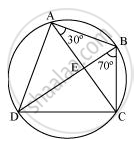# ABCD is a cyclic quadrilateral whose diagonals intersect at a point E. If ∠DBC = 70°, ∠BAC is 30°, find ∠BCD. Further, if AB = BC, find ∠ECD. - Mathematics

ABCD is a cyclic quadrilateral whose diagonals intersect at a point E. If ∠DBC = 70°, ∠BAC is 30°, find ∠BCD. Further, if AB = BC, find ∠ECD.

#### SolutionFor chord CD,

∠CBD = ∠CAD (Angles in the same segment)

∠BCD + 100° = 180°

∠BCD = 80°

In ΔABC,

AB = BC (Given)

∴ ∠BCA = ∠CAB (Angles opposite to equal sides of a triangle)

⇒ ∠BCA = 30°

We have, ∠BCD = 80°

⇒ ∠BCA + ∠ACD = 80°

30° + ∠ACD = 80°

⇒ ∠ACD = 50°

⇒ ∠ECD = 50°

Is there an error in this question or solution?
Chapter 10: Circles - Exercise 10.5 [Page 185]

#### APPEARS IN

NCERT Class 9 Maths
Chapter 10 Circles
Exercise 10.5 | Q 6 | Page 185

Share